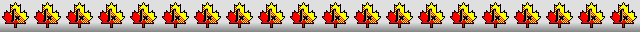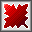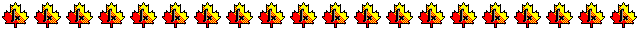Maple worksheets on the derivation of order 10 Runge-Kutta schemesDerivation of 18 stage, order 10 Runge-Kutta schemes - RKcoeff10a.mws

• Curtis' scheme and a modification of his scheme.
See:
"High-order Explicit Runge-Kutta Formulae, Their uses, and Limitations", by A.R.Curtis,
Journal of the Institute of Mathematics and its Applications, Vol. 21 (1978) , pages 47 to 59.

• Coefficients for the schemes and some properties
RKcoeff10b_1.pdf     RKcoeff10b_2.pdf

Derivation of an embedded order 8 scheme for an order 10 Runge-Kutta method - RKcoeff10a_(8)2.mws

• An embedded order 8 scheme for a modification of Curtis' scheme

• Coefficients for the combined scheme and some properties
RKcoeff10a(8)_2.pdf

Derivation of 17 stage, order 10 Runge-Kutta schemes - RKcoeff10b.mws

• Hairer's scheme and some modifications of his scheme.
See:
"A Runge-Kutta Method of Order 10", by E. Hairer,
Journal of the Institute of Mathematics and its Applications (1975) 16, pages 35 to 55.

• Coefficients for Ernst Hairer's scheme and some properties
RKcoeff10b_1.pdf

Derivation of an embedded order 8 scheme for an order 10 Runge-Kutta method - RKcoeff10b_(8).mws

• An embedded order 8 scheme for Ernst Hairer's scheme

• Coefficients for the scheme and some properties
RKcoeff10b(8)_1.pdf

Derivation of embedded order 6 and 9 schemes for an order 10 Runge-Kutta method - RKcoeff10b_(6).mws     RKcoeff10b_(9).mws

• Defective embedded order 6 and 9 schemes for Ernst Hairer's order 10 scheme

Derivation of 21 stage combined order 10 and 9 Runge-Kutta schemes - RKcoeff10c.mws   RKcoeff10d.mws

Derivation of 17 stage, order 10 Runge-Kutta schemes - RKcoeff10e.mws

• Terry Feagin's scheme and some modifications of his scheme.

• Coefficients for two of the schemes and some properties
RKcoeff10e_1.pdf     RKcoeff10e_2.pdf

Derivation of embedded order 8 schemes for order 10 Runge-Kutta methods - RKcoeff10e_(8)1.mws   RKcoeff10e_(8)2.mws

• Embedded order 8 schemes for Terry Feagin's scheme and a modification of his scheme

• Coefficients for combined schemes and some properties
RKcoeff10e(8)_1.pdf     RKcoeff10e(8)_2.pdf

Derivation of embedded order 8 and 9 schemes for an order 10 Runge-Kutta method - RKcoeff10e_(8,9).mws

• Defective embedded order 8 and 9 schemes for Terry Feagin's order 10 scheme

Derivation of 17 stage, order 10 Runge-Kutta schemes - RKcoeff10f.mws

• Hiroshi Ono's scheme and some modifications of his scheme.
See: "A Runge-Kutta method of order 10 which minimizes truncation error", by Hiroshi Ono, Ibaraki University,
The Japan Society for Industrial and Applied Mathematics, Vol. 13, No. 1, 2003, pages 35 to 44.

• Coefficients for two of the schemes and some properties
RKcoeff10f_1.pdf     RKcoeff10f_2.pdf

Derivation of embedded order 8 schemes for order 10 Runge-Kutta methods -
RKcoeff10f_(8)1.mws
RKcoeff10f_(8)2.mws   RKcoeff10f_(8)3.mws   RKcoeff10f_(8)4.mws

Derivation of 21 stage combined order 10 and 9 Runge-Kutta schemes - RKcoeff10g.mws

Derivation of 22 stage combined order 10 and 9 Runge-Kutta schemes -
RKcoeff10h.mws
RKcoeff10k.mws     RKcoeff10m.mws     RKcoeff10n.mws     RKcoeff10p.mws

Derivation of 18 stage order 10 Runge-Kutta schemes and order 9 embedded schemes -
RKcoeff10q.mws
RKcoeff10r.mws          RKcoeff10s.mws

RKcoeff10q_(9).mws    RKcoeff10r_(9).mws     RKcoeff10s_(9).mws

Procedures related to the construction of Runge-Kutta schemes - butcher.zip

Procedures for root-finding and determining minimum points  - roots.zip

Procedures for numerical integration  - intg.zip

Top of page

Main index